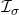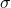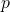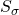# Asymptotically ideal invariant equivalence

### Full PDFcreative_2018_27_2_215_220

In this paper, the concepts of asymptotically-equivalence,-asymptotically equivalence, st\-rong\-ly-asymptotically equivalence and strongly-asymptotically-equivalence for real number sequences are de\-fi\-ned. Also, we give relationships among these new type equivalence concepts and the concept of \linebreak-asymptotically equivalence which is studied in [Savaş, E. and Patterson, R. F.,-asymptotically lacunary statistical equivalent sequences, Cent. Eur. J. Math., 4 (2006), No. 4, 648–655].